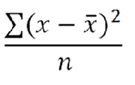# 參考資料：基本函數

## 絕對值 (列)

``````ABS(metric)
``````

## 欄最大值

``````MAXV(metric)
``````

## 欄最小值

``````MINV(metric)
``````

## 欄總和

``````SUM(metric)
``````

## 計數 (表格)

``````COUNT(metric)
``````

## 指數 (列)

``````EXP(metric)
``````

## 乘冪

``````pow(x,y) = x<sup>y</sup> = x*x*x*… (y times)
``````

## 平均值 (表格)

``````MEAN(metric)
``````

## 中位數 (表格)

``````MEDIAN(metric)
``````

## 模數

col1 / col2 的餘數，使用歐幾里德輾轉相除法。

``````x = floor(x/y) + modulo(x,y)
``````

``````modulo(4,3) = 1
modulo(-4,3) = -1
modulo(-3,3) = 0
``````

``````modulo(modulo(x,y)+y,y)
``````

## 百分位數 (表格)

``````PERCENTILE(metric,k)
``````

k 0 至 100 (含) 範圍內的百分位數值。

## 四分位數 (表格)

``````QUARTILE(metric,quart)
``````

quart 指出傳回哪個值*。

*如果 quart = 0，QUARTILE 會傳回最小值。如果 quart = 1，QUARTILE 會傳回第一個四分位數 (第 25 個百分位數)。如果 quart = 2，QUARTILE 會傳回第一個四分位數 (第 50 個百分位數)。如果 quart = 3，QUARTILE 會傳回第一個四分位數 (第 75 個百分位數)。如果 quart = 4，QUARTILE 會傳回最大值。

## 四捨五入

``````ROUND(metric)
``````

``````round( 314.15, 0) = 314
round( 314.15, 1) = 314.1
round( 314.15, -1) = 310
round( 314.15, -2) = 300
``````

## 平方根 (列)

``````SQRT(metric)
``````

## 標準差 (表格)

STDEV 的方程式為：``````STDEV(metric)
``````

## 變異數 (表格)

VARIANCE 的方程式為：``````VARIANCE(metric)
``````

1. 將數字減去平均值。
2. 取結果的平方。
3. 將其加入總計。

1

2

3